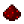# vectorA:crossFunction vector:cross Returns the vector which resulted in the cross product of the two vectors. Syntax vector:cross(vector vect) Returns number Part of ComputerCraft API vector

## ExamplesExample Creates a cross product of two vectors and prints the resulting vector. Code ```local a = vector.new(1, 2, 3) local b = vector.new(4, 5, 6) local c = a:cross(b) print(c.x) print(c.y) print(c.z) --(-3) --6 --(-3) ```Example Code to recreate the function. Gives insight into what the function does. Code ```local a = vector.new(1, 2, 3) local b = vector.new(2, 1, 2) local c = vector.new( (a.y * b.z - a.z * b.y), (a.z * b.x - z.x * b.z), (a.x * b.y - a.y * b.x)) --c.x = 1 --c.y = 4 --c.z = -3 ```

It is important to note that a:cross(b) will not usually equal b:cross(a).